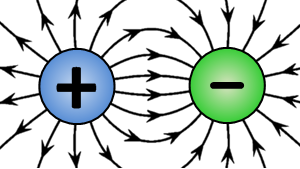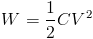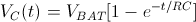# Electric Charge

## What is electric charge?

Electric charge is a fundamental physical property of matter. Electric charge can be positive or negative. Matter repels other matter of the same charge and attracts other matter having the opposite charge. The unit used for electric charge is a Coulomb [C]. While the exact nature of charge is still unknown at a fundamental level, it is generally accepted to represent a specific state of matter which cannot be explained at the current level of scientific knowledge.

Electric charge is quantized, meaning that charge can only have discrete values. An elementary charge is denoted as e, and approximately equals 1.602·10-19 C. The electron bears a charge of -e and it is a negatively charged particle. In contrast, a proton is a positively charged particle, bearing a charge of +e.

An intuitive way to understand the quantized nature of charge is to imagine an electrically neutral object as a box containing an equal number of protons (positive charges) and electrons (negative charges). Protons are fixed and cannot be taken out or added to the box. Since the number of protons and electrons is equal, the total sum of the electric charge inside the box is zero for electrically neutral objects.In order to make the object negatively charged, the only way to do so is to somehow add more electrons into the box. As electrons are indivisible particles, it is only possible to add an integer number of electrons - one cannot add half an electron into the box. As a result, the total charge of the object is N times the charge of a single electron, which equals -e·N, where N is an integer number. Similarly, in order to make an object positively charged, it is necessary to remove N electrons from the box and the resulting total charge of the object is e·N in this case.

## Net charge of an object

One way for an object to become electrically charged is to rub an object with another dissimilar object. This effect has been known since ancient times. For example, rubbing a glass rod with a piece of wool will result in the glass rod being positively charged, while the piece of wool will end up with a negative charge. This is known as the triboelectric effect, which uses friction to transfer free electrons from the glass rod to the wool piece. Since electrons are not created or destroyed in the process, the number of electrons leaving the glass rod is equal to the number of electrons which arrive at the piece of wool. As a result, the charge of the glass rod is equal in value to the charge of the piece of wool, but of opposite sign. Another way to charge an object is by applying an electric current using a power source.

## Charging a capacitor

Capacitors are devices which are designed to store electric energy by accumulating electric charge on their electrodes. In a circuit, the charge leaves one electrode of the capacitor and arrives at the other electrode. Therefore, the amount of charge on one electrode is equal in value to the amount of charge on the other electrode, but of opposite sign. The more charge a capacitor stores, the more energy is stored on the capacitor, according to the formula:where W is the energy stored, C is the capacitance and V is the voltage across the capacitor.

To understand the charging process, consider a completely discharged capacitor as the starting point. In order to charge a capacitor, a power source is connected to its terminals. The moment power is applied to the capacitor, the power source, for example a battery, begins to force electrons to move from one electrode of the capacitor to the other. As electrons start accumulating on one electrode, the voltage across the capacitor increases. Electrons keep accumulating on the electrode of the capacitor which is connected to the negative terminal of the battery until the voltage across the capacitor is equal to the voltage across the battery. This process is not linear - the voltage across the capacitor increases in an exponential manner. The equation for the voltage across the capacitor terminals as a function of time is:where VC(t) is the voltage across the capacitor as a function of time, VBAT is the battery voltage, R is the series resistance in the circuit and C is the capacitance. The nature of R comes from the internal resistance of the battery combined with the ESR of the capacitor and any additional resistors wired in series between the battery and the capacitor.